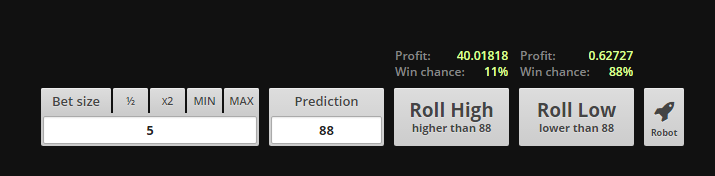# I ask for the presence of a Math God!

## Recommended Posts

Hello Math Gods of phpfreaks.

I am in need of a .... formula....

Let's inspect the outcomes..As we can see, we have the BET SIZE (5), PREDICTION (88) which gives us our profits 40.01818 and 0.62727

So my question is: how do we get the Roll High Profits(40.01818), and Roll Low Profits(0.62727) from BET SIZE and PREDICTION?

Anyone got a php function/formula for this?

Or just a general math formula? (for a live example visit: https://rollin.io)

##### Share on other sites

Those aren't the numbers I'm seeing.

It should be basic gambling math: expected profit = bet * odds of losing / odds of winning.

##### Share on other sites

Those aren't the numbers I'm seeing.

It should be basic gambling math: expected profit = bet * odds of losing / odds of winning.

Yes, my head hurts after using lots of math, but here lies to key:

1.  namespace("Rollin.Casino.Dice.Stats", function() {
2.     var t, e;
3.     return t = namespace.use("Rollin.Casino.Dice.Dice"), e = function() {
4.         function e(t, e) {
5.             this.number = t, this.dice = e
6.         }
7.         return e.prototype.decimal = 5, e.prototype.getData = function(e, n, r) {
8.             var i, o, s, a, u;
9.             switch (> t.MAX_NUMBER - 1 && (= t.MAX_NUMBER - 1), r < t.MIN_NUMBER + 1 && (= t.MIN_NUMBER + 1), i = this.dice.getFee(),n) {
10.                 case t.PREDICTION_BIGGER:
11.                     o = (100 - i) / (t.MAX_NUMBER - r), s = t.MAX_NUMBER - r;
12.                     break;
13.                 default:
14.                     o = (100 - i) / r, s = r
15.             }
16.             return a = e * o, u = a - e, a > 99999 && (= 99999), u > 99999 && (= 99999), a = this.number.floorDecimal(a, this.decimal), u= this.number.floorDecimal(u, this.decimal), this.number.isNaN(a) && (= 0), this.number.isNaN(u) && (= 0), {
17.                 multiplier: o,
18.                 odds: s,
19.                 payout: a,
20.                 profit: u
21.             }
22.         }, e
23.     }()
24. }),

I just can't seem to quite figure out the correct formula

##### Share on other sites

I just can't seem to quite figure out the correct formula

Well then, good luck. I'm sure you'll eventually arrive at the formula I told you.

 Maybe you're getting thrown off because your numbers are wrong? When I enter 5/88 on their site I get 40/0.625. Not the 40.01818/0.62727 in your screenshot. I don't know how you got those but they're wrong.

It's also possible you didn't notice that the random number range is 0-99 and your choice must be 1-98; that's where the house advantage comes in.

[edit 2] And one more thing: the odds of winning/losing in the formula do not include the... I don't know what it's called, but they don't include the number that you automatically lose with. Since you always lose only on your own number, odds of winning + odds of losing = 99%. Yeah, math.

Edited by requinix

##### Share on other sites

Well then, good luck. I'm sure you'll eventually arrive at the formula I told you.

 Maybe you're getting thrown off because your numbers are wrong? When I enter 5/88 on their site I get 40/0.625. Not the 40.01818/0.62727 in your screenshot. I don't know how you got those but they're wrong.

It's also possible you didn't notice that the random number range is 0-99 and your choice must be 1-98; that's where the house advantage comes in.

[edit 2] And one more thing: the odds of winning/losing in the formula do not include the... I don't know what it's called, but they don't include the number that you automatically lose with. Since you always lose only on your own number, odds of winning + odds of losing = 99%. Yeah, math.

I think our numbers are different because there are "levels" based on how much you have wagered. For instance I am level 3, and my house edge is only 0.96%. I believe level 1 (your level)  house edge is 0.99%.

##### Share on other sitesThis topic is now closed to further replies.

×

• #### Activity

• Chat
×
• Create New...